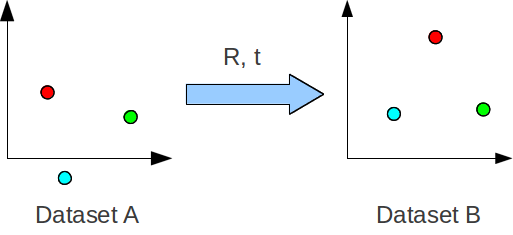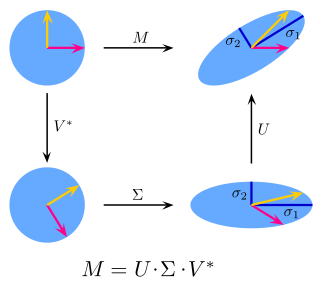Go to Practical Course

# Linear Algebra. Points matching with SVD in 3D space

If something moved it doesn't mean that it changedWe need to find the best rotation & translation params between two sets of points in 3D space. This type of transformation called Euclidean as it preserves sizes.Our task to solve the equation

\begin{align}R*A + t = B\end{align}

## Solution

There are many ways of getting things done almost in any case, but currently we will use SVD for this. You ask why? — Because the matrix is basically a transformation and with SVD can be decomposed on Rotation(U), Scaling(Σ), Rotation(V) (in special case when A is mxm matrix, but this gives us a clue. deeper explanation you can find in paper Least-Squares Rigid Motion Using SVD)Rotation

To get rotation first we need to find out the center of it. So we will find centroids of two datasets.

\begin{align}centroid_p = mean([{x_i,y_i,z_i} \in X])\end{align}

First, we will re-center our datasets and create covariance matrix H of two them, where Pa, Pb are points in datasets.

\begin{align}H = \sum _{i=1}^N{(P_A^i-centroid_A)}{(P_B^i-centroid_B)}^T\end{align}

Now we use SVD to find out U and V matrices

\begin{align}[U,S,V] = \mathrm{SVD}(H)\end{align}

And our rotation matrix are equal

\begin{align}R = V*U.T\end{align}

### Problem with SVD

Because solving SVD implies some sort of randomness(there can be a different number of correct solutions) it sometimes makes a rotation matrix to be sign reflected.
We can fix this by checking the determinant of R matrix and if it negative then multiply 3rd column of R by -1

Translation

This is very simple.

1. recenter with -centroid_A
2. Rotate and move to the center of dataset B\begin{align}t = -R*centroid_A + centroid_B\end{align}

### References

1. Least-Squares Rigid Motion Using SVD  [PDF]
Olga Sorkine-Hornung and Michael Rabinovich, 2017. Department of Computer Science, ETH Zurich.
2. Singular value decomposition  [HTML]
Wikipedia
3. Singular value decomposition and principal component analysis  [HTML]
Wall, Michael E.; Rechtsteiner, Andreas; Rocha, Luis M., 2002. Los Alamos National Laboratory.